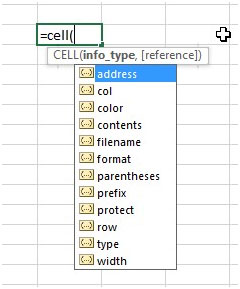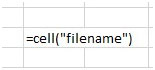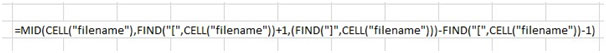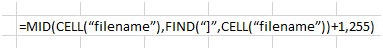# Cell Function Returns Sheet Name, Workbook Name and Path in Microsoft Excel 2010

We have a useful function in excel called as the CELL function which can be modified to return the sheet name, workbook name and the file path.  In this article we will learn how to return name of the excel sheet. Lets see how this is done.

Considering that we want this info for the current file.  First lets understand the CELL Function.  The syntax is =CELL(info_type, [reference]).  The CELL Function will return information about the formatting, location or contents of the 1st cell in the reference.  The info_type can be the filename, address, color, etc.  There is an available list of options in the dropdown which appears so you can choose the required one.  The reference is the current workbook which you are using.The file in this example is located at “B:\”. To obtain the file path, workbook name and sheet name , we useThis will give us the output as B:\[Book1.xlsm]Sheet2 which is the file path + workbook name + sheet name of the workbook in this example.

Now lets move one step ahead.  To get the file path only from B:\[Book1.xlsm]Sheet2, we use=MID(CELL(“filename”),1,FIND(“[“,CELL(“filename”))-1)

The highlighted section will be evaluated first which will find the location of the opening box bracket “[“ in the function. It finds it as location 4.

Our function then narrows down to =MID(CELL(“filename”),1,3).  We need the characters starting from the 1st character to the 3rd character (4-1=3) using the mid function.  So the output we get will be the file path without the workbook name.   And we get the result as “B:\”

Now lets derive the workbook name.  Our function isWe need to derive the workbook name from this result B:\[Book1.xlsm]Sheet2. Lets understand how it works.

=MID(CELL(“filename”),,FIND(“[",CELL("filename"))+1,(FIND("]“,CELL(“filename”)))-FIND(“[“,CELL(“filename”))-1)

The highlighted portion will be evaluated first.  The workbook name is always in box brackets so we have to search for the opening box bracket [ and closing box bracket ] and our result is within these 2.  So this part of the function will return the starting position of the text.

=MID(CELL(“filename”),FIND(“[",CELL("filename"))+1,(FIND("]“,CELL(“filename”)))-FIND(“[“,CELL(“filename”))-1)

This highlighted portion will evaluate to the last parameter of the MID function which is the number of characters to be extracted from the starting position.  So it calculates the position of the ending box bracket which is 15 in this case and deducts the position of the starting box bracket which is 4 and another -1 to exclude the starting box bracket.  So the mid function is complete as =MID(CELL(“filename”),5,10) and we get the result as Book1.xlsm as per our example.

Now lets derive the last aspect which is the sheet name.  Our function isIt’s a very simple function.  First, the highlighted section in this function is evaluated - =MID(CELL(“filename”),,FIND(“]”,CELL(“filename”))+1,255)

This will give us the positing of the ending box bracket + 1 (to exclude the box bracket.  So in our example, this results in 15+1 = 16.  We directly arrive at the MID function now.  It looks like

=MID(CELL(“filename”),16,255).  We are asking the function to give us 255 (which is the maximum characters a cell can hold) characters starting from the 16th character of the filename.

So the result we get is Sheet2 as in this example.

To conclude, we can use the same base function =CELL(“filename”) with some enhancements to derive the information we require about the file name.

In this way we can get worksheet name in cell with CELL function.

1.Try this function if the other isn't working for you:

=RIGHT(CELL("filename"),LEN(CELL("filename"))-FIND("]",CELL("filename")))

2."Thank you for your information, I found it very useful. There is small error in this formula. ""Worksheet function that returns the workbook name:""

The corrected one should read as follow

=MID(CELL(""filename""),FIND(""["",CELL(""filename""))+1,FIND(""]"",CELL(""filename""))-FIND(""["",CELL(""filename""))-1) "

3."the function =MID(CELL(""filename""),FIND(""]"",CELL(""filename""))+1,255) returns the same value on each sheet unless you add a cell reference after ""filename"".

the formula should look like this when done:
=MID(CELL(""filename"",b1),FIND(""]"",CELL(""filename"",b1))+1,255)

By adding a cell reference after filename, the value returned should be the worksheet name of the proper worksheet and not the same value for all worksheets."

4.The functions listed here return same value if these are used in more than one worksheet or workbook...

5.I can't get this formula to work, but this formula has 8 functions in the argument, I thought you could only have 7 functions in the formula.

6.the function =MID(CELL("filename"),FIND("]",CELL("filename"))+1,255) seems to be a global function. Putting multiple instaces of this function on separte sheets, always returns the same name

7."The following function works correctly to return the workbook name:
=MID(CELL(""filename""),FIND(""["",CELL(""filename""))+1,(FIND(""]"",CELL(""filename""))+1)-FIND(""["",CELL(""filename""))-2)"

8.The formula listed here: =MID(CELL("filename"),1,FIND("[",CELL("filename"))+1, FIND("]",(CELL("filename"))-FIND("[",CELL("filename"))-1) seems to be missing a closing parenthesis.

9."the function =MID(CELL(""filename""),FIND(""]"",CELL(""filename""))+1,255) returns the same value on each sheet unless you add a cell reference after ""filename"".
the formula should look like this when done:
=MID(CELL(""filename"",b1),FIND(""]"",CELL(""filename"",b1))+1,255)
By adding a cell reference after filename, the value returned should be the worksheet name of the proper worksheet and not the same value for all worksheets."

10.The functions listed here return same value if these are used in more than one worksheet or workbook.

11.I can't get this formula to work, but this formula has 8 functions in the argument, I thought you could only have 7 functions in the formula.

12.the function =MID(CELL("filename"),FIND("]",CELL("filename"))+1,255) seems to be a global function. Putting multiple instaces of this function on separte sheets, always returns the same name

13.The following function works correctly to return the workbook name: =MID(CELL("filename"),FIND("[",CELL("filename"))+1,(FIND("]",CELL("filename"))+1)-FIND("[",CELL("filename"))-2)

14.The formula listed here: =MID(CELL("filename"),1,FIND("[",CELL("filename"))+1, FIND("]",(CELL("filename"))-FIND("[",CELL("filename"))-1) seems to be missing a closing parenthesis.

Terms and Conditions of use

The applications/code on this site are distributed as is and without warranties or liability. In no event shall the owner of the copyrights, or the authors of the applications/code be liable for any loss of profit, any problems or any damage resulting from the use or evaluation of the applications/code.